# Hilbert transform

of a functionThe improper integral

 $$g\left(x\right) = \frac{1}{\pi} \int_{0}^{\infty}\frac{f\left(x+t\right)-f\left(x-t\right)}{t}\mathrm{d}t$$. (1)

If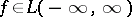, the functionexists for almost-all values of. If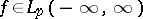,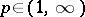, the functionalso belongs to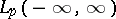and the inversion formula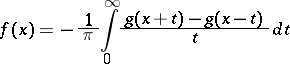(2)

is valid almost-everywhere. Here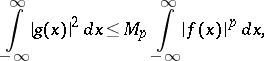(3)

where the constantdepends only on.

Formulas (1) and (2) are equivalent to the formulas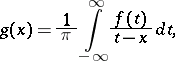(4)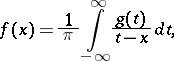(5)

in which the integrals are understood in the sense of the principal value.

The integral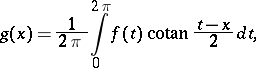(6)

understood in the sense of its principal value, is also called the Hilbert transform of. This integral is often called the Hilbert singular integral. In the theory of Fourier series the functiondefined by (6) is said to be conjugate with.

If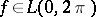,exists almost-everywhere, and ifsatisfies a Lipschitz condition of order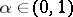,exists for anyand satisfies the same condition. If,, thenhas the same property, and an inequality analogous to (3) in which the integrals are taken over the interval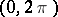is valid. Thus, the integral operators generated by the Hilbert transform are bounded (linear) operators on the respective spaces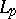.

Ifsatisfies a Lipschitz condition, or if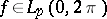, and also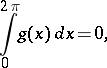the following inversion formula is valid: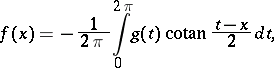(7)

and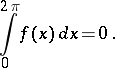In the class of functions which satisfy a Lipschitz condition, equation (7) is valid everywhere, and in the class of functions with integrable-th power, it is valid almost-everywhere.

Each one of the pairs of formulas written above, such as (4) or (5), may be considered as an integral equation of the first kind, and the second formula yields the solution of this equation.

If the functionsand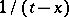are considered as kernels of integral operators, they are often referred to as the Hilbert kernel and as the Cauchy kernel. In the case of the unit circle, there exists a simple relationship between these kernels: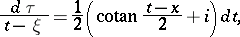where,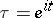.